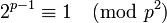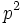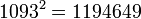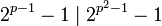Square of Wieferich prime is Poulet number

Statement

Supposeis a Wieferich prime, i.e., a prime number such that:Then,is a Poulet number (also called Sarrus number), i.e., a Fermat pseudoprime to base 2.

Particular cases

There are only two known Wieferich primes: 1093 and 3511. Hence, this fact gives only two Poulet numbers:and.

Proof

Given:is a prime such thatTo prove:Proof: We have:Thus,divides. This gives that:Combining this with the given information, we get that.